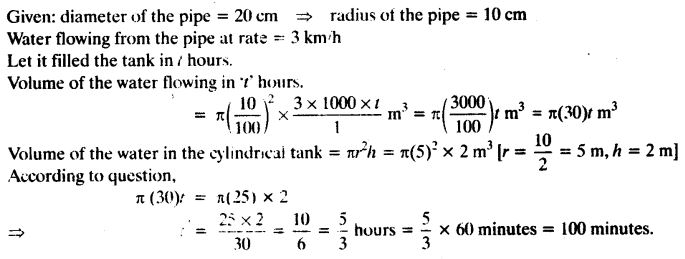ncert textbook

# NCERT Solutions for Class 10 Maths Chapter 13 Surface Areas and Volumes Ex 13.3

Get Free NCERT Solutions for Class 10 Maths Chapter 13 Ex 13.3 PDF. Surface Areas and Volumes Class 10 Maths NCERT Solutions are extremely helpful while doing your homework. Exercise 13.3 Class 10 Maths NCERT Solutions were prepared by Experienced ncert-books.in Teachers. Detailed answers of all the questions in Chapter 13 Maths Class 10 Surface Areas and Volumes Exercise 13.3 provided in NCERT TextBook.

NCERT Solutions for Class 10 Maths Chapter 13 Surface Areas and Volumes Ex 13.3 are part of NCERT Solutions for Class 10 Maths. Here we have given NCERT Solutions for Class 10 Maths Chapter 13 Surface Areas and Volumes Ex 13.3

Question 1.
A metallic sphere of radius 4.2 cm is melted and recast into the shape of a cylinder of radius 6 cm. Find the height of the cylinder.
Solution: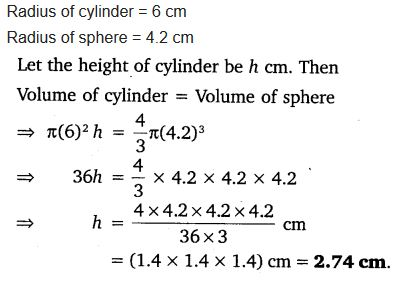Question 2.
Metallic spheres of radii 6 cm, 8 cm and 10 cm, respectively, are melted to form a single solid sphere. Find the radius of the resulting sphere.
Solution: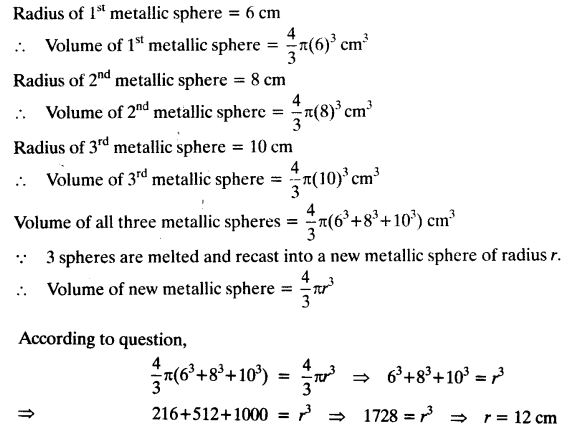Question 3.
A 20 m deep well with diameter 7 m is dug and the earth from digging is evenly spread out to form a platform 22 m by 14 m. Find the height of the platform.
Solution: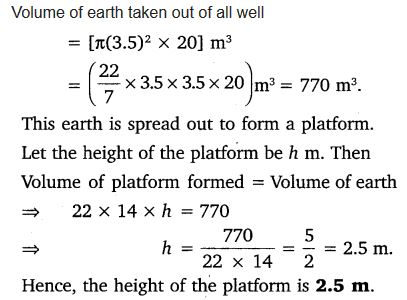Question 4.
A well of diameter 3 m is dug 14 m deep. The earth taken out of it has been spread evenly all around it in the shape of a circular ring of width 4 m to form an embankment. Find the height of the embankment.
Solution:Question 5.
A container shaped like a right circular cylinder having diameter 12 cm and height 15 cm is full of ice cream. The ice cream is to be filled into cones of height 12 cm and diameter 6 cm, having a hemispherical shape on the top. Find the number of such cones which can be filled with ice cream.
Solution:Question 6.
How many silver coins, 1.75 cm in diameter and of thickness 2 mm, must be melted to form a cuboid of dimensions 5.5 cm × 10 cm × 3.5 cm?
Solution:Question 7.
A cylindrical bucket, 32 cm high and with radius of base 18 cm, is filled with sand. This bucket is emptied on the ground and a conical heap of sand is formed. If the height of the conical heap is 24 cm, find the radius and slant height of the heap.
Solution:Question 8.
Water in a canal, 6 m wide and 1.5 m deep, is flowing with a speed of 10 km/h. How much area will it irrigate in 30 minutes, if 8 cm of standing water is needed?
Solution: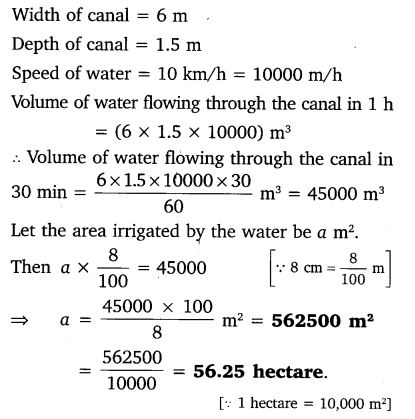Question 9.
A farmer connects a pipe of internal diameter 20 cm from a canal into a cylindrical tank in her field, which is 10 m in diameter and 2 m deep. If water flows through the pipe at the rate of 3 km/h, in how much time will the tank be filled?
Solution: